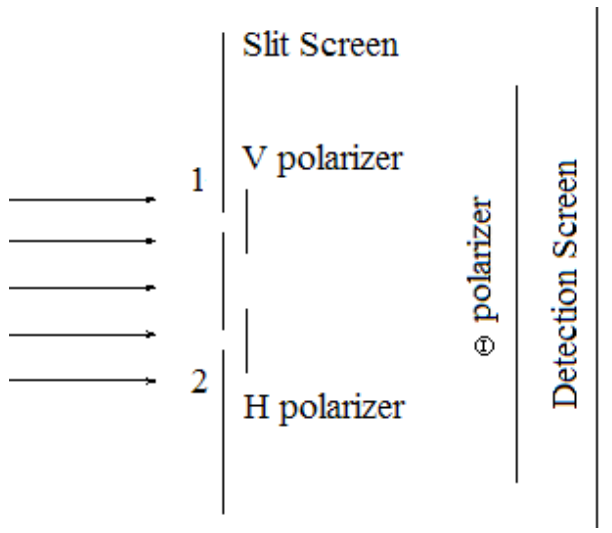# 1.48: Which‐way Markers and Post‐selection in the Double‐slit Experiment

$$\newcommand{\vecs}{\overset { \rightharpoonup} {\mathbf{#1}} }$$ $$\newcommand{\vecd}{\overset{-\!-\!\rightharpoonup}{\vphantom{a}\smash {#1}}}$$$$\newcommand{\id}{\mathrm{id}}$$ $$\newcommand{\Span}{\mathrm{span}}$$ $$\newcommand{\kernel}{\mathrm{null}\,}$$ $$\newcommand{\range}{\mathrm{range}\,}$$ $$\newcommand{\RealPart}{\mathrm{Re}}$$ $$\newcommand{\ImaginaryPart}{\mathrm{Im}}$$ $$\newcommand{\Argument}{\mathrm{Arg}}$$ $$\newcommand{\norm}{\| #1 \|}$$ $$\newcommand{\inner}{\langle #1, #2 \rangle}$$ $$\newcommand{\Span}{\mathrm{span}}$$ $$\newcommand{\id}{\mathrm{id}}$$ $$\newcommand{\Span}{\mathrm{span}}$$ $$\newcommand{\kernel}{\mathrm{null}\,}$$ $$\newcommand{\range}{\mathrm{range}\,}$$ $$\newcommand{\RealPart}{\mathrm{Re}}$$ $$\newcommand{\ImaginaryPart}{\mathrm{Im}}$$ $$\newcommand{\Argument}{\mathrm{Arg}}$$ $$\newcommand{\norm}{\| #1 \|}$$ $$\newcommand{\inner}{\langle #1, #2 \rangle}$$ $$\newcommand{\Span}{\mathrm{span}}$$$$\newcommand{\AA}{\unicode[.8,0]{x212B}}$$

This tutorial examines the real reason which‐way information destroys the double‐slit diffraction pattern and how the so‐called ʺquantum eraserʺ restores it. The double‐slit experiment is presented schematically below.The wave function for a photon illuminating the slit screen is written as a superposition of the photon being present at both slits simultaneously.

$|\Psi\rangle=\frac{1}{\sqrt{2}}\left[\left|x_{1}\right\rangle+\left|x_{2}\right\rangle\right] \nonumber$

Assuming initially infinitesimally thin slits, the diffraction pattern is calculated by projecting this superposition into momentum space.

$\langle p | \Psi\rangle=\frac{1}{\sqrt{2}}\left[\left\langle p | x_{1}\right\rangle+\left\langle p | x_{2}\right\rangle\right]=\frac{1}{\sqrt{2}}\left[\frac{1}{\sqrt{2 \pi}} \exp \left(-i p x_{1}\right)+\frac{1}{\sqrt{2 \pi}} \exp \left(-i p x_{2}\right)\right] \nonumber$

Assuming slits of finite width, $$\delta$$, positioned as indicated below, the momentum wave function becomes,

 Position of first slit: $$x_{1} :=0$$ Position of second slit: $$x_{2} :=1$$ Slit width: $$\delta :=0.2$$

$\Psi (p) : = \frac{\int_{x_{1} -\frac{\delta}{2}}^{x_{1}+\frac{\delta}{2}} \frac{1}{\sqrt{2 \cdot \pi}} \cdot \exp (-i \cdot p \cdot x) \cdot \frac{1}{\sqrt{\delta}} d x+\int_{x_{2}- \frac{\delta}{2}}^{x_{2}+\frac{\delta}{2}} \frac{1}{\sqrt{2 \cdot \pi}} \cdot \exp (-i \cdot p \cdot x) \cdot \frac{1}{\sqrt{\delta}} d x}{\sqrt{2}} \nonumber$

The double‐slit diffraction pattern is the momentum distribution function.When polarization markers are attached to the slits we have the following schematic of the double‐slit experiment with which‐way information.According to the Encyclopedia Britannica, Fresnel and Arago ʺusing an apparatus based on Youngʹs [double‐slit] experimentʺ observed that ʺtwo beams polarized in mutually perpendicular planes never yield fringes.ʺ We now look at the quantum mechanical explanation of this phenomena. Fresnel and Arago, working during the 19th Century, provided a valid classical explanation.

The coordinate and momentum wave functions now become,

$|\Psi\rangle=\frac{1}{\sqrt{2}}\left[\left|x_{1}\right\rangle|\mathrm{V}\rangle+\left|x_{2}\right\rangle|H\rangle\right] \quad \text{where} \; |\mathrm{V}\rangle=\left(\begin{array}{l}{1} \\ {0}\end{array}\right) \qquad|\mathrm{H}\rangle=\left(\begin{array}{l}{0} \\ {1}\end{array}\right) \nonumber$

$\Psi_{\mathrm{hv}}(\mathrm{p}) :=\frac{1}{\sqrt{2}} \int_{\mathrm{x}_{1}-\frac{\delta}{2}}^{\mathrm{x}_{1}+\frac{\delta}{2}} \frac{1}{\sqrt{2 \cdot \pi}} \exp (-\mathrm{i} \cdot \mathrm{p} \cdot \mathrm{x}) \cdot \frac{1}{\sqrt{\delta}} \mathrm{d} \mathrm{x} \cdot\left(\begin{array}{l}{1} \\ {0}\end{array}\right) +\frac{1}{\sqrt{2}} \cdot \int_{x_{2} \frac{\delta}{2}}^{x_{2}+\frac{\delta}{2}} \frac{1}{\sqrt{2 \cdot \pi}}\cdot \exp (-i \cdot p \cdot x) \cdot \frac{1}{\sqrt{\delta}} d x\cdot\left(\begin{array}{l}{0} \\ {1}\end{array}\right) \nonumber$

This leads to the following momentum distributions at the detection screen.The usual explanation for this effect is that it is now possible to know which slit the photons went through, and that such knowledge destroys the interference fringes because the photons are no longer in a superposition of passing through both slits, but rather a mixture of passing through each slit half the time.

However, a more reasonable explanation is that the tags are orthogonal polarization states, and because of this the interference (cross) terms in the momentum distribution, $$\left|\Psi_{\mathrm{hy}}(\mathrm{p})\right|^{2}$$, vanish leaving a pattern at the detection screen which is the sum of two single‐slit diffraction patterns, one from the upper slit and the other from the lower slit.

That this is a reasonable analysis is confirmed when the so‐called quantum eraser, a $$\theta$$ polarizer, is placed before the detection screen as diagramed below.The presence of the $$\theta$$ polarizer is represented mathematically by: $$\langle\theta|=(\cos (\theta) \quad \sin (\theta))$$

Projection of $$\Psi_{hv}$$(p) onto $$\langle \theta |$$ accounts for the action of the $$\theta$$ polarizer, yielding the momentum wave function after the polarizer.

$\Psi^{\prime}(\mathrm{p}, \theta)=\left(\begin{array}{c}{\cos (\theta)} \\ {\sin (\theta)}\end{array}\right)^{\mathrm{T}} \cdot \Psi_{\mathrm{hv}}(\mathrm{p}) \nonumber$

If the $$\theta$$ polarizer is oriented at any angle other than 0 or multiples of $$\frac{\pi}{2}$$, the interference fringes reappear to some degree. As an example the diffraction pattern observed for a angles of $$\pm \frac{\pi}{4}$$ are shown along with the results from the previous graphs.A common explanation for the reappearance of the interference fringes is that the $$\frac{\pi}{4}$$ polarizers have erased the which‐way information. A less esoteric explanation is achieved by recognizing that |H > and |V > are even superpositions of |D > (D = diagonal = $$\frac{\pi}{4}$$) and |A > (A = anti‐diagonal = $$-\frac{\pi}{4}$$), and that the probability that |H > and |V > photons will pass a diagonal polarizer is 0.5. It also easily explains the phase shift that is observed with the anti‐diagonal ($$-\frac{\pi}{4}$$) ʺeraser.ʺ

$|\mathrm{V}\rangle=\frac{1}{\sqrt{2}}[|\mathrm{D}\rangle+|\mathrm{A}\rangle]=\frac{1}{\sqrt{2}}\left[\frac{1}{\sqrt{2}}\left(\begin{array}{l}{1} \\ {1}\end{array}\right)+\frac{1}{\sqrt{2}}\left(\begin{array}{c}{1} \\ {-1}\end{array}\right)\right] \nonumber$

$|\mathrm{H}\rangle=\frac{1}{\sqrt{2}}[|\mathrm{D}\rangle-|\mathrm{A}\rangle]=\frac{1}{\sqrt{2}}\left[\frac{1}{\sqrt{2}}\left(\begin{array}{l}{1} \\ {1}\end{array}\right)-\frac{1}{\sqrt{2}}\left(\begin{array}{c}{1} \\ {-1}\end{array}\right)\right] \nonumber$

This is an example of post‐selection. After passing the slit screen with its polarization markers (state preparation), but before the detection screen (measurement), a subset of photon states is selected by the orientation of the $$\theta$$ polarizer, say |D> or |A>. After these polarizers the photons are in one of the following polarized superpositions.

$|\Psi\rangle=\frac{1}{2}\left[\left|x_{1}\right\rangle|\mathrm{D}\rangle+\left|x_{2}\right\rangle|\mathrm{D}\rangle\right] \text { or }|\Psi\rangle=\frac{1}{2}\left[\left|x_{1}\right\rangle|\mathrm{A}\rangle-\left|x_{2}\right\rangle|\mathrm{A}\rangle\right] \nonumber$

Projecting these states into momentum space assuming finite slit widths yields the reduced diffraction patterns shown in the figure above. The following probability calculations support the arguments presented here.

$\int_{-\infty}^{\infty}(|\Psi(\mathrm{p})|)^{2} \mathrm{dp} \text { float, } 2 \rightarrow 1.0 \nonumber$

$\int_{-\infty}^{\infty}\left(\left|\Psi_{\mathrm{hv}}(\mathrm{p})\right|\right)^{2} \mathrm{dp} \text { float, } 2 \rightarrow 1.0 \nonumber$

$\int_{-\infty}^{\infty}\left(\left|\Psi^{n}\left(\mathrm{p}, \frac{\pi}{4}\right)\right|\right)^{2} \text { dp float, } 2 \rightarrow 0.50 \nonumber$

$\int_{-\infty}^{\infty}\left(\big|\Psi^{r}\left(\mathrm{p},-\frac{\pi}{4}\right)\big|\right)^{2} \text { dp float, } 2 \rightarrow 0.50 \nonumber$

If which‐path erasure was really occurring the last two integrals would equal 1.0

This page titled 1.48: Which‐way Markers and Post‐selection in the Double‐slit Experiment is shared under a CC BY 4.0 license and was authored, remixed, and/or curated by Frank Rioux via source content that was edited to the style and standards of the LibreTexts platform; a detailed edit history is available upon request.